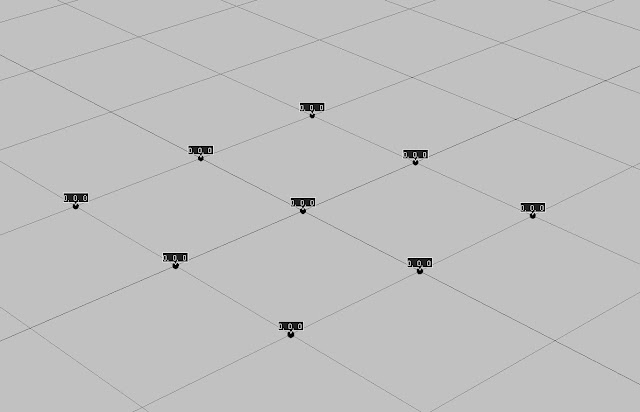## Functions

#### Functions represent relationships between variables. They take an input variable, manipulate it in some way and produce an output variable.*click to make bigger*
The Input(X) can also be called: domain, domain elements, independent variable or argument.
The output(x+4) can also be called: range, image, range elements, dependent variables or value of function.
The relationship between (X) and x + 4 is F

Taking f(x) = x + 4 into ICE would look like the image below if f(x) = y.#### *click to make bigger*

Before I applied the Function to a grid pointcloud it looked like the image below. All the points are at there initial position.  All the points read as 0,0,0 position, this is local position coordinates for every point, in the global coordinate space all the points except the middle point would have either 1 or -1 for its Z and X value. *The global value of right corner point would read as: -1 (X), 0 (Y), 1 (Z)*#### *click to make bigger*

When I apply the function to the grid pointcloud it looks like the image below. Note how it added the global value of X not the local value. Then the + 4 giving us a graph that increases linearly in space. *You can see the global coordinate in the image below*#### |cos(x+z)| ^ 10/2

| cos(x^2+z^2) | ^ (1/2)

cos( |x| + |z| ) * ( x + z )

y = |cos(x) + cos(z)| ^ (1/2)

| cosx^2+cosz^2) | ^ (1/2)